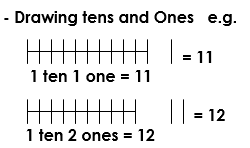# PLACE VALUES-p.1

##### This unit includes tens and the ones,how they are used and represented on the abacus

Lesson: 1– The ones have only one figure or digit. (e.g. 3, 5, 6, etc…)

– We don’t tie a bundle when the sticks are less than ten.– We tie a bundle when the sticks are ten (10)

– The Tens have 2 figures or digits. (e.g. 10, 30 40, 71, etc…)

Activity:

Practical lesson – counting and tying bundles of tens using sticks / straws

Lesson: 3

Tens and OnesActivity: Drawing tens and ones

a)10 =_______________       f. 20 =______________

b)25 =_______________     g. 19 = _____________

c)34 =_______________     h.   8=______________

d)12 = _______________     i. 60 = _____________

e)42 = _______________     j. 93 = _____________

Lesson: 4

Tens and Ones

Filling in tens and ones e.g.

24 = _____ tens _____ ones

____ = 5 tens 7 ones

Lesson:5

Tens and Ones

• The abacus
• Representing numbers on the abacus. E.g.
••  Drawing and filling the abacus

SEE ALL
•YOU